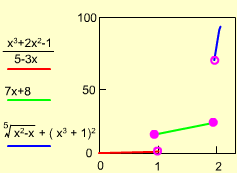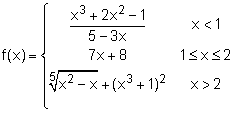Ch 1. Limits Multimedia Engineering Math Limit of aSequence Limit of aFunction LimitLaws Continuity RateChange
 Chapter 1. Limits 2. Derivatives I 3. Derivatives II 4. Mean Value 5. Curve Sketching 6. Integrals 7. Inverse Functions 8. Integration Tech. 9. Integrate App. 10. Parametric Eqs. 11. Polar Coord. 12. Series Appendix Basic Math Units Search eBooks Dynamics Fluids Math Mechanics Statics Thermodynamics Author(s): Hengzhong Wen Chean Chin Ngo Meirong Huang Kurt Gramoll ©Kurt GramollMATHEMATICS - CASE STUDY IntroductionPiecewise f(x) function diagram In a series of Aluminum cutting experiments, Jimmy summarizes the relationship between the force and the heat conducted to the cutter. He finds that the heat is a piecewise function of the force. In order to amylases the property of the cutter, he wants to analysis the heat released to the cutter at jump points. The unit for the force is kN, and the unit for the heat is J. What is known: The function is:Questions Analysis the heat conducted to the cutter when force is 1 and 2 kN. Approach f(x) is a piecewise continuous function, the quantity of f(x) when x = 1- and x = 1+ are different, same thing for x = 2- and x= 2+.